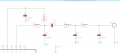# Working out total Inductance and Capacitance

#### abc14

Joined Oct 15, 2017
123
Just wanted to validate the vaules I worked out total Inductance and Capacitance are correct.For total Inductance I think L1 and L2 are parallel hence it would be L1*L2/L1+L2 = 155/4.7+33 = 4.11

And L3 and L4 are series to L1||L2 hence 4.11 + 6.8 + 6.8 = 17.71 nH

And for Capacitance.. C2 and C3 are in series hence it will be 47*5.6/47+5.6 = 4.91 and C5 and C7 are in parrallel hence it will be 4.91 + 8.2 + 6.8 = 19.91

Hence Ltotal= 17.71 nH
and Ctotal = 19.91 pF

#### Papabravo

Joined Feb 24, 2006
14,239
Because the components interact with each other, the circuit above will not behave the same as two lumped components with the values you have calculated. It looks like a multi-pole bandpass filter

#### abc14

Joined Oct 15, 2017
123

#### Papabravo

Joined Feb 24, 2006
14,239
View attachment 176578

Yes its acting as filter for 868Mhz rf ICs, but I cannot figure out how they setting up required frequency of 868 mhz with this circuit.
The methods for setting up such a filter are derived from the requirements. The methods are detailed in a college text like the following:

https://www.amazon.com/Analog-Filter-Design-Van-Valkenburg/dp/0030592461/ref=sr_1_fkmrnull_2?crid=GTMAETWPRPQ0&keywords=analog+filter+design+valkenburg&qid=1556980549&s=books&sprefix=Analog+filter+Design,aps,247&sr=1-2-fkmrnull

As for reverse engineering the filter, I would try simulation in LTSpice as a first step.
https://www.analog.com/en/design-center/design-tools-and-calculators/ltspice-simulator.html